# http www comp nus edu sgcs 1010 Unit

• Slides: 33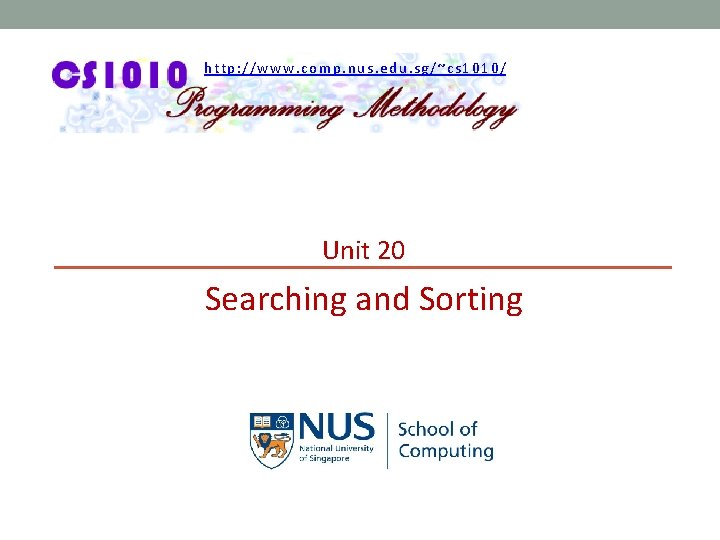http: //www. comp. nus. edu. sg/~cs 1010/ Unit 20 Searching and SortingAaron Tan, NUS Searching and Sorting Unit 20 - 2 Unit 20: Searching and Sorting Objectives: § Understand the basic searching algorithms and § § sorting algorithms Introduce the concept of complexity analysis (informally) Implement the searching and sorting algorithms using arrays Reference: § Chapter 7 Array Pointers § Section 7. 6 Searching and Sorting an ArrayAaron Tan, NUS Searching and Sorting Unit 20 - 3 Unit 20: Searching and Sorting (1/2) 1. Overall Introduction 2. Introduction to Searching 3. Linear Search § § Demo #1 Performance 4. Binary Search SEARCHINGAaron Tan, NUS Searching and Sorting Unit 20 - 4 Unit 20: Searching and Sorting (2/2) 5. Introduction to Sorting 6. Selection Sort § § Demo #2 Performance 7. Bubble Sort § § Demo #3 Performance 8. More Sorting Algorithms 9. Animated Sorting Algorithms SORTING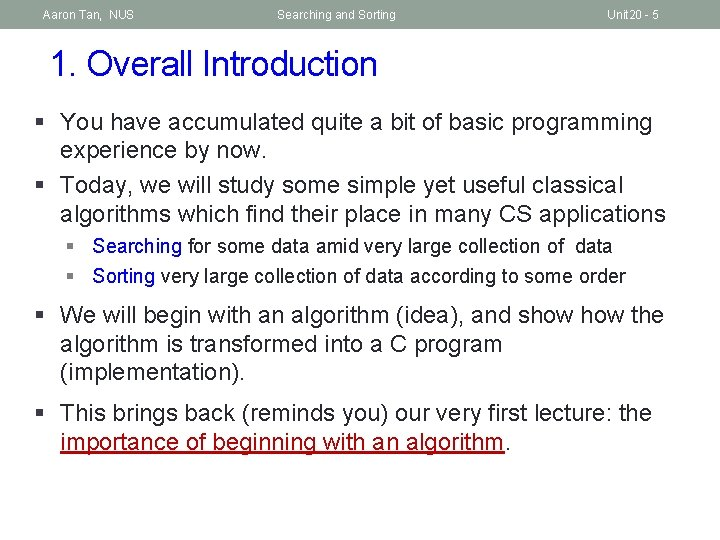Aaron Tan, NUS Searching and Sorting Unit 20 - 5 1. Overall Introduction § You have accumulated quite a bit of basic programming experience by now. § Today, we will study some simple yet useful classical algorithms which find their place in many CS applications § Searching for some data amid very large collection of data § Sorting very large collection of data according to some order § We will begin with an algorithm (idea), and show the algorithm is transformed into a C program (implementation). § This brings back (reminds you) our very first lecture: the importance of beginning with an algorithm.Aaron Tan, NUS Searching and Sorting Unit 20 - 6 2. Introduction to Searching (1/2) § Searching is a common task that we carry out without much thought everyday. § § Searching for a location in a map Searching for the contact of a particular person Searching for a nice picture for your project report Searching for a research paper required in your course § In this lecture, you will learn how to search for an item (sometimes called a search key) in an array.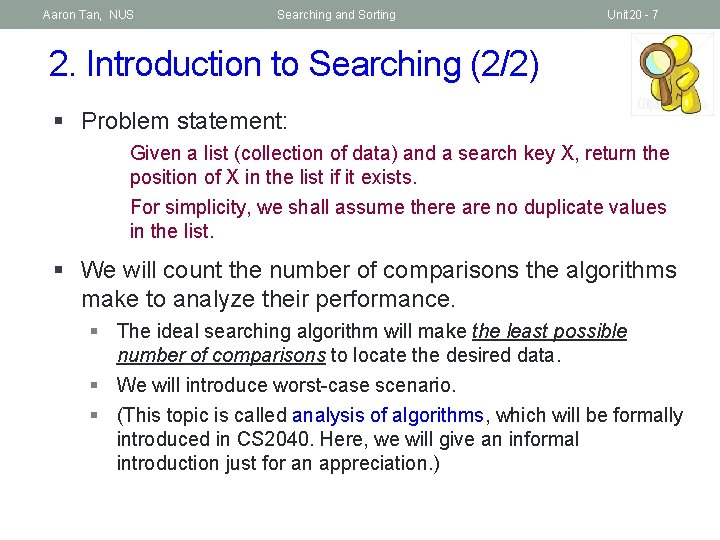Aaron Tan, NUS Searching and Sorting Unit 20 - 7 2. Introduction to Searching (2/2) § Problem statement: Given a list (collection of data) and a search key X, return the position of X in the list if it exists. For simplicity, we shall assume there are no duplicate values in the list. § We will count the number of comparisons the algorithms make to analyze their performance. § The ideal searching algorithm will make the least possible number of comparisons to locate the desired data. § We will introduce worst-case scenario. § (This topic is called analysis of algorithms, which will be formally introduced in CS 2040. Here, we will give an informal introduction just for an appreciation. )Aaron Tan, NUS Searching and Sorting Unit 20 - 8 3. Linear Search (1/3) § Also known as Sequential Search § Idea: Search the list from one end to the other end in linear progression. § Algorithm: // Search for key in list A with n items linear_search(A, n, key) { for i = 0 to n-1 if Ai is key then report i } Example: Search for 24 in this list 87 12 51 9 24 63 no yes! no no no § Question: What to report if key is not found? § Aim for a clean design Return 4Aaron Tan, NUS Searching and Sorting Unit 20 - 9 3. Linear Search: Demo #1 (2/3) § If the list is an array, how would you implement the Linear Search algorithm? // To search for key in arr using linear search // Return index if found; otherwise return -1 int linear. Search(int arr[], int size, int key) { int i; See linear_search. c for full program } for (i=0; i<size; i++) if (key == arr[i]) return i; return -1; Useful and common technique § Question: What if array contains duplicate values of the key? Index of the first element found will be returned.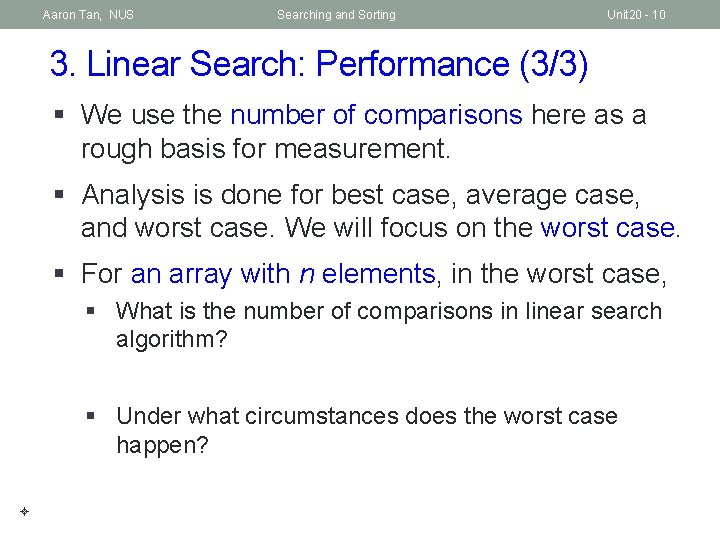Aaron Tan, NUS Searching and Sorting Unit 20 - 10 3. Linear Search: Performance (3/3) § We use the number of comparisons here as a rough basis for measurement. § Analysis is done for best case, average case, and worst case. We will focus on the worst case. § For an array with n elements, in the worst case, § What is the number of comparisons in linear search algorithm? § Under what circumstances does the worst case happen?Aaron Tan, NUS Searching and Sorting Unit 20 - 11 4. Binary Search (1/6) § You are going to witness a radically different approach, one that has become the basis of many well-known algorithms in Computer Science! § The idea is simple and fantastic, but when applied on the searching problem, it has this pre-condition that the list must be sorted before-hand. § How the data is organized (in this case, sorted) usually affects how we choose/design an algorithm to access them. § In other words, sometimes (actually, very often) we seek out new way to organize the data so that we can process them more efficiency. More of this in CS 2040 Data Structures and Algorithms.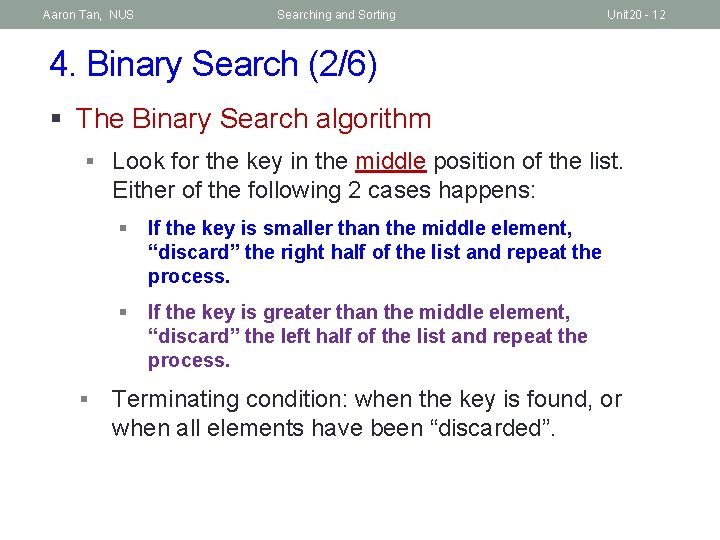Aaron Tan, NUS Searching and Sorting Unit 20 - 12 4. Binary Search (2/6) § The Binary Search algorithm § Look for the key in the middle position of the list. Either of the following 2 cases happens: § § If the key is smaller than the middle element, “discard” the right half of the list and repeat the process. § If the key is greater than the middle element, “discard” the left half of the list and repeat the process. Terminating condition: when the key is found, or when all elements have been “discarded”.Aaron Tan, NUS Searching and Sorting Unit 20 - 13 4. Binary Search (3/6) § Example: Search key = 23 arr  5         12 17 23 38 44 77 84 90 Found! Return 3 1. low = 0, high = 8, mid = (0+8)/2 = 4 arr = 38 > 23 2. low = 0, high = 3, mid = (0+3)/2 = 1 arr = 12 < 23 3. low = 2, high = 3, mid = (2+3)/2 = 2 arr = 17 < 23 4. low = 3, high = 3, mid = (3+3)/2 = 3 arr = 23 == 23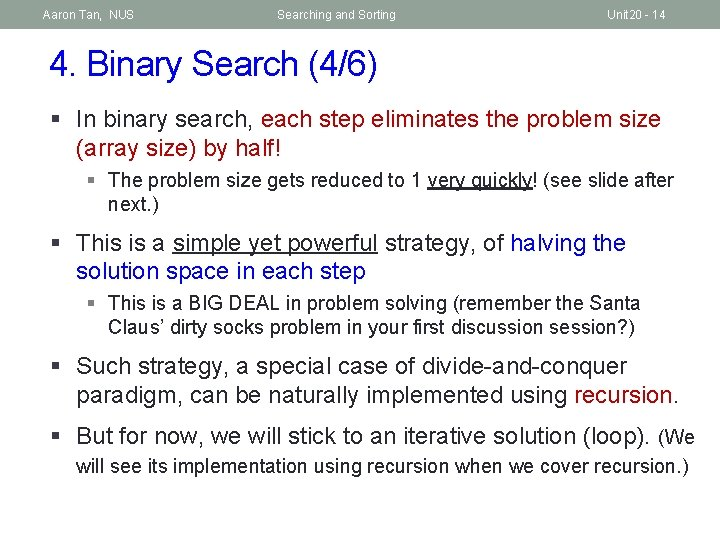Aaron Tan, NUS Searching and Sorting Unit 20 - 14 4. Binary Search (4/6) § In binary search, each step eliminates the problem size (array size) by half! § The problem size gets reduced to 1 very quickly! (see slide after next. ) § This is a simple yet powerful strategy, of halving the solution space in each step § This is a BIG DEAL in problem solving (remember the Santa Claus’ dirty socks problem in your first discussion session? ) § Such strategy, a special case of divide-and-conquer paradigm, can be naturally implemented using recursion. § But for now, we will stick to an iterative solution (loop). (We will see its implementation using recursion when we cover recursion. )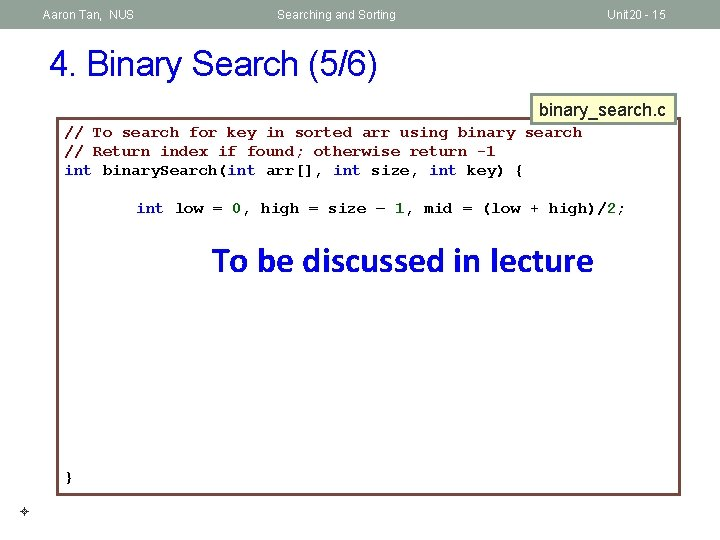Aaron Tan, NUS Searching and Sorting Unit 20 - 15 4. Binary Search (5/6) binary_search. c // To search for key in sorted arr using binary search // Return index if found; otherwise return -1 int binary. Search(int arr[], int size, int key) { int low = 0, high = size – 1, mid = (low + high)/2; To be discussed in lecture }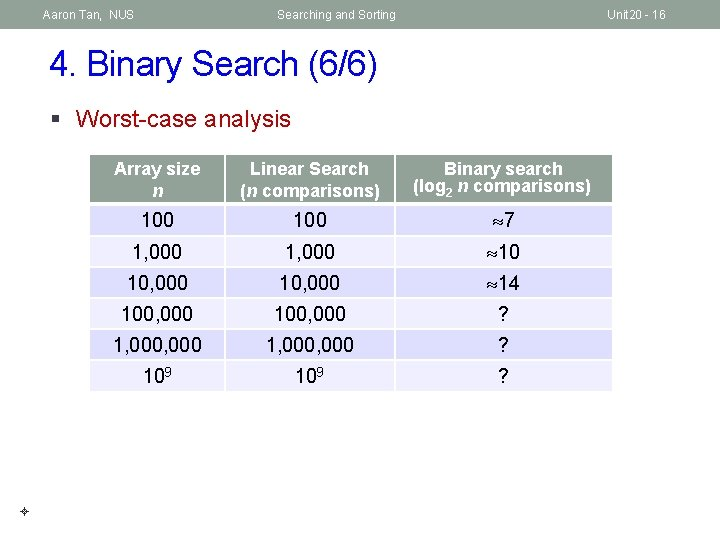Aaron Tan, NUS Searching and Sorting Unit 20 - 16 4. Binary Search (6/6) § Worst-case analysis Array size n Linear Search (n comparisons) Binary search (log 2 n comparisons) 100 7 1, 000 10 10, 000 14 100, 000 ? 1, 000, 000 ? 109 ?Aaron Tan, NUS Searching and Sorting Unit 20 - 17 5. Introduction to Sorting (1/2) § Sorting is any process of arranging items in some sequence and/or in different sets – Wikipedia. § Sorting is important because once a set of items is sorted, many problems (such as searching) become easy. § Searching can be speeded up. (From linear search to binary search) § Determining whether the items in a set are all unique. § Finding the median item in a list. § Many others…Aaron Tan, NUS Searching and Sorting Unit 20 - 18 5. Introduction to Sorting (2/2) § Problem statement: Given a list of n items, arrange the items into ascending order. § We will implement the list as an integer array. § We will introduce two basic sort algorithms. § We will count the number of comparisons the algorithms make to analyze their performance. § The ideal sorting algorithm will make the least possible number of comparisons to arrange data in a designated order. § We will compare the algorithms by analyzing their worstcase performance.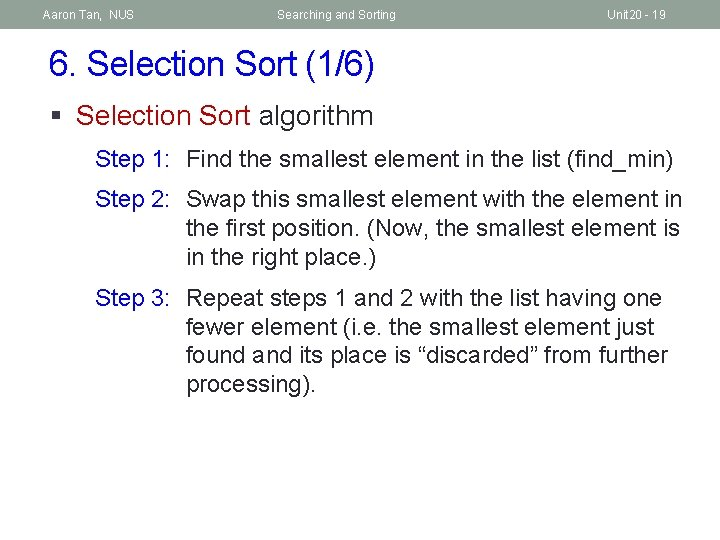Aaron Tan, NUS Searching and Sorting Unit 20 - 19 6. Selection Sort (1/6) § Selection Sort algorithm Step 1: Find the smallest element in the list (find_min) Step 2: Swap this smallest element with the element in the first position. (Now, the smallest element is in the right place. ) Step 3: Repeat steps 1 and 2 with the list having one fewer element (i. e. the smallest element just found and its place is “discarded” from further processing).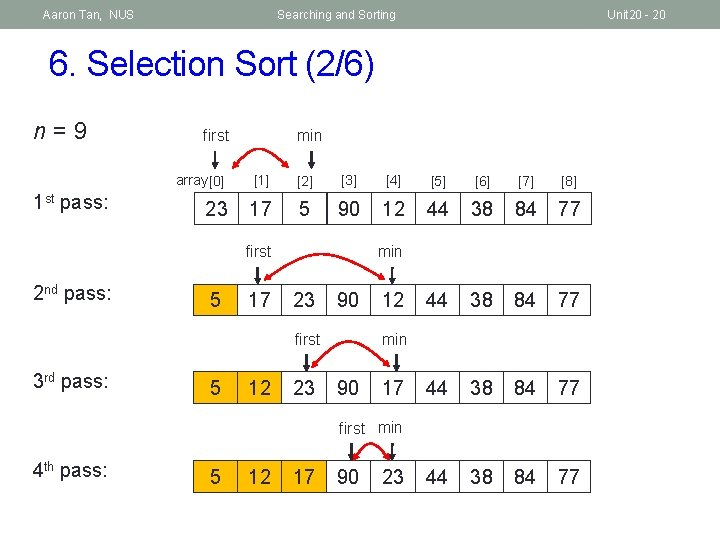Aaron Tan, NUS Searching and Sorting Unit 20 - 20 6. Selection Sort (2/6) n=9 1 st pass: first min array          23 17 5 90 12 44 38 84 77 min first 2 nd pass: 5 17 23 90 min first 3 rd pass: 5 12 23 12 90 17 first min 4 th pass: 5 12 17 90 23Aaron Tan, NUS Searching and Sorting Unit 20 - 21 6. Selection Sort (3/6) n=9 5 th pass: Q: How many passes for an array with n elements? 5 12 17 23 90 44 first 6 th pass: 5 12 17 23 38 n – 1 passes min first 44 38 84 77 min 90 min first 7 th pass: 5 12 17 23 38 44 90 84 first 77 min 8 th pass: 5 12 17 23 38 44 77 84 90 Final array: 5 12 17 23 38 44 77 84 90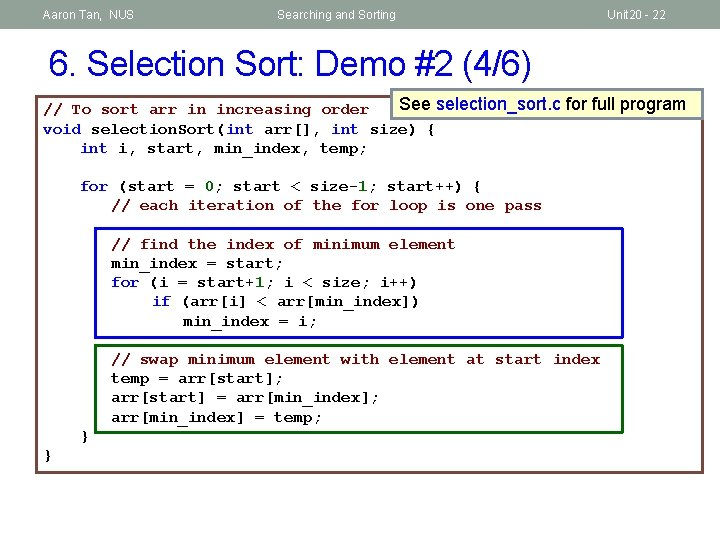Aaron Tan, NUS Searching and Sorting Unit 20 - 22 6. Selection Sort: Demo #2 (4/6) See selection_sort. c for full program // To sort arr in increasing order void selection. Sort(int arr[], int size) { int i, start, min_index, temp; for (start = 0; start < size-1; start++) { // each iteration of the for loop is one pass // find the index of minimum element min_index = start; for (i = start+1; i < size; i++) if (arr[i] < arr[min_index]) min_index = i; // swap minimum element with element at start index temp = arr[start]; arr[start] = arr[min_index]; arr[min_index] = temp; } }Aaron Tan, NUS Searching and Sorting Unit 20 - 23 6. Selection Sort: Performance (5/6) § We choose the number of comparisons as our basis of analysis. § Comparisons of array elements occur in the inner loop, where the minimum element is determined. § Assuming an array with n elements. Table below shows the number of comparisons for each pass. § The total number of comparisons is calculated in the formula below. § Such an algorithm is called an O(n 2) algorithm, or quadratic algorithm, in terms of running time complexity. Pass #comparisons 1 n– 1 2 n– 2 3 n– 3 … … n– 1 1Aaron Tan, NUS Searching and Sorting Unit 20 - 24 6. Selection Sort (6/6) § Selection sort is classified under exchange sort, where elements are exchanged in the process. § We could search for the minimum element as described earlier, or search for the maximum element and exchange it with the last element of the working array (assuming we sort in ascending order).Aaron Tan, NUS Searching and Sorting Unit 20 - 25 7. Bubble Sort (1/5) § Selection sort makes one exchange at the end of each pass. § What if we make more than one exchange during each pass? § The key idea Bubble sort is to make pairwise comparisons and exchange the positions of the pair if they are in the wrong order.Aaron Tan, NUS Searching and Sorting Unit 20 - 26 7. Bubble Sort: One Pass of Bubble Sort (2/5) 0 1 23 17 2 3 5 4 5 6 7 8 90 12 44 38 84 77 17 5 exchange 17 23 5 exchange 90 12 44 38 84 77 17 5 exchange 17 5 23 12 44 38 90 84 77 exchange 23 90 12 44 38 84 77 ok 23 12 44 90 38 84 77 17 5 23 12 44 38 84 90 77 exchange 23 12 90 44 38 84 77 exchange 17 5 23 12 44 38 84 77 90 Q: Is the array sorted? Q: What have we achieved? Done!Aaron Tan, NUS Searching and Sorting Unit 20 - 27 7. Bubble Sort: Demo #3 (3/5) See bubble_sort. c for full program // To sort arr in increasing order void bubble. Sort(int arr[], int size) { int i, limit, temp; for (limit = size-2; limit >= 0; limit--) { // limit is where the inner loop variable i should end for (i=0; i<=limit; i++) { if (arr[i] > arr[i+1]) { // swap arr[i] with arr[i+1] temp = arr[i]; arr[i] = arr[i+1]; arr[i+1] = temp; } }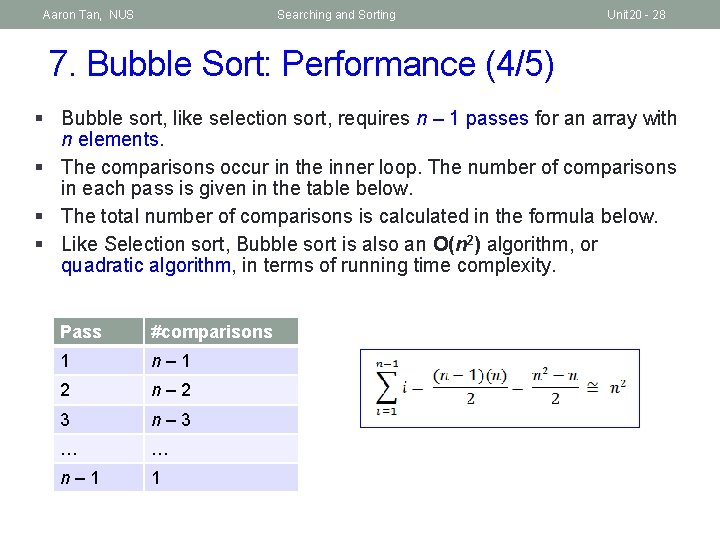Aaron Tan, NUS Searching and Sorting Unit 20 - 28 7. Bubble Sort: Performance (4/5) § Bubble sort, like selection sort, requires n – 1 passes for an array with n elements. § The comparisons occur in the inner loop. The number of comparisons in each pass is given in the table below. § The total number of comparisons is calculated in the formula below. § Like Selection sort, Bubble sort is also an O(n 2) algorithm, or quadratic algorithm, in terms of running time complexity. Pass #comparisons 1 n– 1 2 n– 2 3 n– 3 … … n– 1 1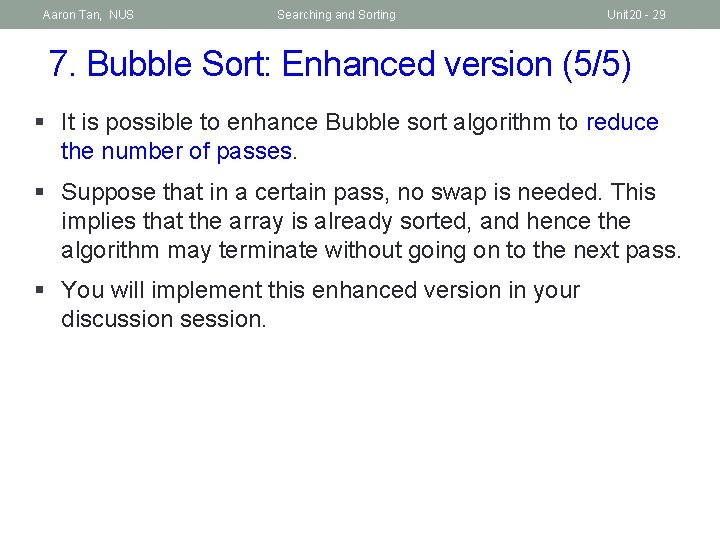Aaron Tan, NUS Searching and Sorting Unit 20 - 29 7. Bubble Sort: Enhanced version (5/5) § It is possible to enhance Bubble sort algorithm to reduce the number of passes. § Suppose that in a certain pass, no swap is needed. This implies that the array is already sorted, and hence the algorithm may terminate without going on to the next pass. § You will implement this enhanced version in your discussion session.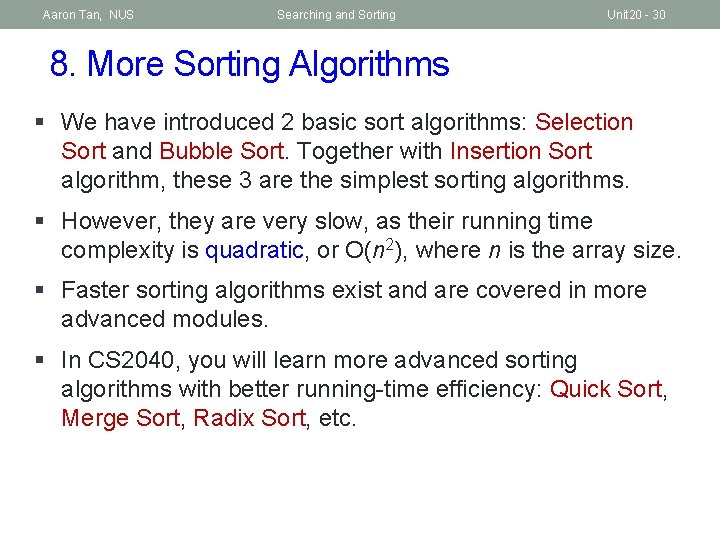Aaron Tan, NUS Searching and Sorting Unit 20 - 30 8. More Sorting Algorithms § We have introduced 2 basic sort algorithms: Selection Sort and Bubble Sort. Together with Insertion Sort algorithm, these 3 are the simplest sorting algorithms. § However, they are very slow, as their running time complexity is quadratic, or O(n 2), where n is the array size. § Faster sorting algorithms exist and are covered in more advanced modules. § In CS 2040, you will learn more advanced sorting algorithms with better running-time efficiency: Quick Sort, Merge Sort, Radix Sort, etc.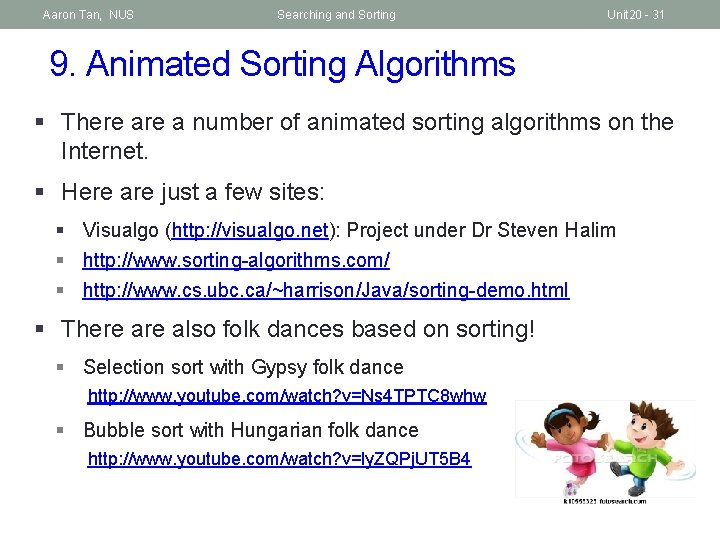Aaron Tan, NUS Searching and Sorting Unit 20 - 31 9. Animated Sorting Algorithms § There a number of animated sorting algorithms on the Internet. § Here are just a few sites: § Visualgo (http: //visualgo. net): Project under Dr Steven Halim § http: //www. sorting-algorithms. com/ § http: //www. cs. ubc. ca/~harrison/Java/sorting-demo. html § There also folk dances based on sorting! § Selection sort with Gypsy folk dance http: //www. youtube. com/watch? v=Ns 4 TPTC 8 whw § Bubble sort with Hungarian folk dance http: //www. youtube. com/watch? v=ly. ZQPj. UT 5 B 4Aaron Tan, NUS Searching and Sorting Unit 20 - 32 Summary n In this unit, you have learned about n 2 search algorithms: Linear (sequential) Search and Binary Search n 2 basic sort algorithms: Selection Sort and Bubble Sort.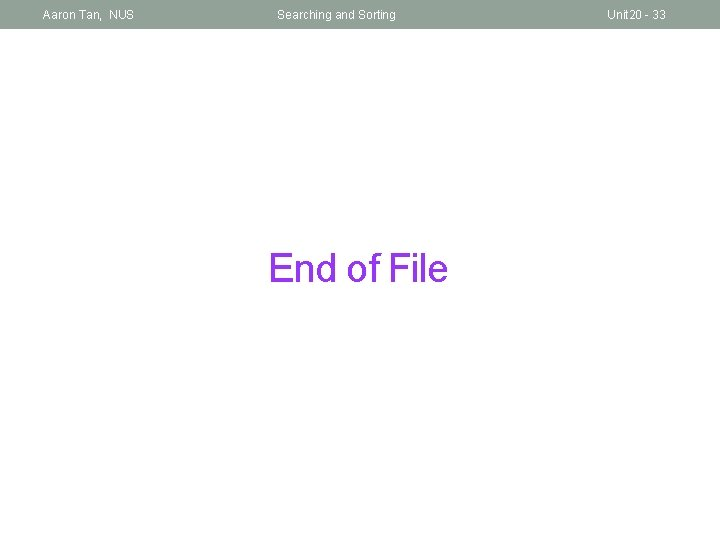Aaron Tan, NUS Searching and Sorting End of File Unit 20 - 33